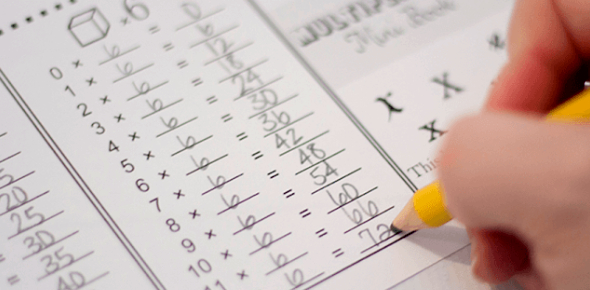# Multiplication Quiz: Ultimate Exam!

57 Questions | Total Attempts: 85Settings.

• 1.
2 x 2 =
• 2.
2 x 3 = or3 x 2 =
• 3.
2 x 4 =or4 x 2 =
• 4.
2 x 5 = or 5 x 2 =
• 5.
2 x 6 =or6 x 2 =
• 6.
2 x 7 =or 7 x 2 =
• 7.
2 x 8 =or8 x 2 =
• 8.
2 x 9 =or 9 x 2 =
• 9.
2 x 11 = or 11 x 2 =
• 10.
2 x 12 =or 12 x 2 =
• 11.
3 x 3 =
• 12.
3 x 4 =or4 x 3 =
• 13.
3 x 5 = or5 x 3 =
• 14.
3 x 6 =or6 x 3 =
• 15.
3 x 7 =or7 x 3 =
• 16.
3 x 8 =or 8 x 3 =
• 17.
3 x 9 =or 9 x 3 =
• 18.
3 x 11 = or 11 x 3 =
• 19.
3 x 12 =or 12 x 3 =
• 20.
4 x 4 =
• 21.
4 x 5 =or 5 x 4 =
• 22.
4 x 6 =or 6 x 4 =
• 23.
4 x 7 =or 7 x 4 =
• 24.
4 x 8 =or 8 x 4 =
• 25.
4 x 9 =or 9 x 4 =
Related TopicsBack to top